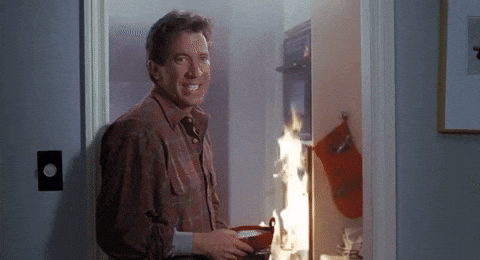# Thanksgiving Gantt Chart

Thanksgiving 🦃 is right around the corner 🎉 – this year we are hosting 17 people 😱. Ingredients:
📦 lubridate
📦 plotly
If you too are hosting way more than your kitchen normally cooks for, perhaps this will be of interest! We decided to make a Google Sheet of the various dishes so we could plot out what will be cooking when.

## Packages we’ll use

library("googlesheets")
library("lubridate")
library("plotly")

## Pull in the data

First we can pull the spreadsheet into R using the googlesheets package.

## Register the google sheet

## Read the sheet into R
dishes_df <- gs_read(dishes_ss)

## Clean up a bit 🛀

dishes_df$start <- as_datetime(dishes_df$start,
tz = "America/Chicago")
dishes_df$finish <- as_datetime(dishes_df$finish,
tz = "America/Chicago")
dishes_df$minutes <- dishes_df$finish - dishes_df$start Let’s pick some lovely turkey-themed colors for our chart 🌈 brought to you by colour lovers. cols <- c("#487878", "#783030", "#904830", "#A87860", "#D89048") dishes_df$color  <- factor(dishes_df$where, labels = cols) ## Order for the chart dishes_df <- dishes_df[order(dishes_df$start,
decreasing = TRUE), ]

## Make the plot 💃

This is inspired by a Plotly blog post!

p <- plot_ly()

for (i in 1:nrow(dishes_df)) {
x = c(dishes_df$start[i], dishes_df$finish[i]),
y = c(i, i),
line = list(color = dishes_df$color[i], width = 20), hoverinfo = "text", text = paste("Dish: ", dishes_df$dish[i],
"<br>",
"Cook time: ",
dishes_df$minutes[i], "minutes<br>", "Where: ", dishes_df$where[i]),
showlegend = FALSE
)
}## Add the dish names to the y-axis, remove grid
p <- layout(p,
xaxis = list(showgrid = FALSE),
yaxis = list(showgrid = FALSE,
tickmode = "array",
tickvals = 1:nrow(dishes_df),
ticktext = unique(dishes_df\$dish)),
margin = list(l = 200, r = 50, b = 50, t = 50, pad = 4),
plot_bgcolor = "#EBE5E5",
paper_bgcolor = "#EBE5E5",
## add a turkey because why not!
images = list(
xref = "paper",
yref = "paper",
x= 0.75,
y= 1,
sizex = 0.25,
sizey = 0.25
) )

)
p

Happy feasting! 🦃❤️Lucy D'Agostino McGowan

Currently excited about: observational study methods, translational research, BB-8